# NCERT Solutions for Class 12 Chemistry Chapter 8 d-and f-Block Elements

In this chapter, we provide NCERT Solutions for Class 12 Chemistry Chapter 8 d-and f-Block Elements for English medium students, Which will very helpful for every student in their exams. Students can download the latest NCERT Solutions for Class 12 Chemistry Chapter 8 d-and f-Block Elements pdf, free NCERT solutions for Class 12 Chemistry Chapter 8 d-and f-Block Elements book pdf download. Now you will get step by step solution to each question. Class 12 Chemistry is a very important subject for entrance exams like IIT JEE, CPMT, etc. NCERT Class 12 chemistry notes in Hindi

## NCERT Solutions for Class 12 Chemistry Chapter 8 The d and f Block Elements

INTEXT Questions

Question 1.
Silver atom has completely filled d-orbitals (4d10) in its ground state. How can you say that it is a transition element?
Solution:
Silver (Z = 47) can exhibit +2 oxidation state wherein it will have incompletely filled d-orbitals (4d), hence it is a transition element.

Question 2.
In the series Sc (Z = 21) to Zn (Z = 30), the enthalpy of atomisation of zinc is the lowest, i.e., 126 kJ mol-1. Why?
Solution:
In the formation of metallic bonds, no electrons from 3d-orbitals are involved in case of zinc, while in all other metals of the 3d-series, electrons from the d-orbitals are always involved in the formation of metallic bonds.

Question 3.
Which of the 3d-series of the transition metals exhibits the largest number of oxidation states and why?
Solution:
Manganese (Z = 25), as its atom has the maximum number of unpaired electrons.

Question 4.
The E°(M2+/M) value for copper is positive (+0.34 V). What is possibly the reason for this? (Hint: consider its high ∆aH° and low ∆hydH°)
Solution:
The high energy to transform Cu(s) to Cu2+(aq) is not found balanced by its hydration energy. Hydration energy and lattice energy of Cu2+ is more than Cu.

Question 5.
How would you account for the irregular variation of ionisation enthalpies (first and second) in the first series of the transition elements?
Solution:
Irregular variation of ionisation enthalpies is mainly attributed to varying degree of stability of different 3d – configurations (e.g., d°, d5, d10 are exceptionally stable).

Question 6.
Why is the highest oxidation state of a metal exhibited in its oxide or fluoride only?
Solution:
Because of small size and high electronegativity oxygen or fluorine can oxidise the metal to its highest oxidation state.

Question 7.
Which is a stronger reducing agent Cr2+ or Fe2+ and why?
Solution:
Cr2+ is stronger reducing agent than Fe2+ Reason: d4 → d3 occurs in case of Cr2+ to Cr2+. But d6 → d5 occurs in case of Fe2+ to Fe2+.
In a medium (like water) d’1 is more stable as compared to d5.

Question 8.
Calculate the ‘spin only’ magnetic moment of M2+(aq) ion (Z = 27).

Solution: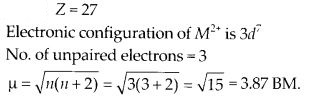Question 9.
Explain why Cu+ ion is not stable in aqueous solutions?
Solution:
Cu+ in aqueous solution undergoes disproportionation, i.e.,
2Cu+(aq) → Cu2+(aq) + Cu(s)
The stability of Cu2+(aq) rather than Cu+(aq) is due to the much more negative ∆hyd H°of Cu2+(aq) than Cu+, which more than compensates for the second ionisation enthalpy of Cu.

Question 10.
Actinoid contraction is greater from element to element than lanthanoid contraction. Why?
Solution:
The 5f electrons are more effectively shielded from nuclear charge. In other words the 5f electrons themselves provide poor shielding from element to element in the seriest.

NCERT Exercises

Question 1.
Write down the electronic configuration of

1. Cr3+
2. Pm3+
3. Cu+
4. Ce4+
5. CO2+
6. Lu2+
7. Mn2+
8. Th4+
9. Cr3+
10. Pm3+
11. Cu+
12. Ce4+
13. CO2+
14. Lu2+
15. Mn2+
16. Th4+

Solution:Question 2.
Why are Mn2+ compounds more stable than Fe2+ towards oxidation of their +3 state?
Solution:
Mn2+ has an electronic configuration of 1s2 2s2 2p6 3s2 3p6 3d5 4s0. To go to Mn3+ state the electron has to be taken out from stable d5 orbital which is half filled and requires very high ionisation energy. In case of Fe2+ ion, the third electron is taken out from 3d6 configuration which results in more stable 3d5 configuration.

Question 3.
Explain briefly how +2 state becomes more and more stable in the first half of the first row With increasing atomic number the transition elements with increasing atomic numbers?
Solution:
With increasing atomic number the effective nuclear charge increases after losing two electrons from s-orbital. The ionic size decreases which results in more stability. The slability is less in the beginning due to too few electrons to lose or share.

Question 4.
To what extent do the electronic configurations decide the stability of oxidation states in the first series of the transition elements? Illustrate your answer with examples.
Solution:
Sc3+ has stable electronic configuration (vacant d-orbital), therefore Sc3+ is more stable than Sc+.

Fe3+ is more stable than Fe2+ due to half filled d-orbitals.

Mn2+ is more stable than Mn3+ due to half filled d-orbitals.

V5+ is more stable (due to vacant d-orbital) than V3+.

Question 5.
What may be the stable oxidation state of the transition element with the following d-electron configurations in the ground state of their atoms: 3d3, 3d5, 3d8 and 3d4 ?
Solution:

1. 3d3 : Stable oxidation state will be +5 due to outer electronic configuration 3d34s2 (+2, +3, +4, +5).
2. 3d5: Stable oxidation state will be +2 and +7 due to outer electronic configuration 3d4s2 (+2, +3, +4, +6, +7).
3. 3d8: Stable oxidation state will be +2 due to outer electronic configuration 3d8 4s2 (+2, +3, +4).
4. 3d4 : Stable oxidation state will be +3 and +6 due to outer electronic configuration 3d4s1. There is no d4 configuration in ground state, as it becomes 3d4s1

Question 6.
Name the oxometal anions of the first series of the transition metals in which the metal exhibits the oxidation state equal to its group number.
Solution:
Manganese shows oxidation state of +7 in its oxometal anion MnO4 which is equivalent to its group number 7. Cr in Cr2O72- and CrO42- show oxidation state +6 which is equivalent to its group number 6.

Question 7.
What is lanthanoid contraction? What are the consequences of lanthanoid contraction?
Solution:
The overall decrease in atomic and ionic radii from lanthanum to lutetium is a unique feature in the chemistry of the lanthanoids. It has far reaching consequences in the chemistry of the third transition series of the elements.

This contraction is attributed to the imperfect shielding of one electron by another in the same sub-shell. However, the shielding of one 4f electron by another is less than one d electron by another with the increase in nuclear charge along the series. There is fairly regular decrease in the sizes with increasing atomic number.

The cumulative effect of the contraction of the lanthanoid series, known as lanthanoid contraction, causes the radii of the members of the third transition series to be very similar to those of the corresponding members of the second series. The almost identical radii of Zr (160 pm) and Hf (159 pm), a consequence of the lanthanoid contraction, account for their occurrence together in nature and for the difficulty faced in their separation.

Question 8.
What are the characteristics of the transition elements and why are they called transition elements? Which of the d-block elements may not be regarded as the transition elements?
Solution:
General characteristics of transition elements.

1. Electronic configuration – (n – 1) d1-10 ns1-2
2. Metallic character – With the exceptions of Zn, Cd and Hg, they have typical metallic structures.
3. Atomic and ionic size – Ions of same charge in a given series show progressive decrease in radius with increasing atomic number.
4. Oxidation state – Variable ; ranging from + 2 to + 7.
5. Paramagnetism – The ions with unpaired electrons are paramagnetic.
6. Ionisation enthalpy – Increases due to increase in molecular charge.
7. Formation of coloured ions – Due to unpaired electrons.
8. Formation of complex compounds – Due to small size and high charge density of metal ions.
9. They possess catalytic properties – Due to their ability to adopt multiple oxidation states.
10. Formation of interstitial compounds.
11. Alloy formation.

They are called transition elements due to their incompletely filled d-orbitals in ground state or any stable oxidation state and they are placed between s and p-block elements. Zn, Cd and Hg have fully filled d° configuration in their ground state hence may not be regarded as the transition elements.

Question 9.
In what way is the electronic configuration of the transition elements different from that of the non-transition elements?.
Solution:
The electronic configuration of the transition elements is (n – 1 )d1-10 ns1-2. (n-1) stands for penultimate shell and d-orbitals may have one to ten d electrons and n denotes valence s or the outermost shell which can have one or two electrons. Hence the basic difference in electronic configuration of transition metals is that their penultimate shell is incomplete and progressively filled and not the valence shell.

Question 10.
What are the different oxidation states exhibited by the lanthanoids?
Solution:
In lanthanoids +3 oxidation state is predominant. However occasionally +2 and +4 ions in the solution or in solid compounds are also obtained, e.g. Ce4+, Tb4+, Eu2+, Yb2+, etc. +2 and +4 oxidation states are exhibited due to extra stability of empty, half – filled or fully filled f – subshells.

Question 11.
Explain giving reasons :

1. Transition metals and many of their compounds show paramagnetic behaviour.
2. The enthalpies of atomisation of the transition metals are high.
3. The transition metals generally form coloured compounds.
4. Transition metals and their many compounds act as good catalysts.

Solution:
(i) Paramagnetism arises from the presence of unpaired electrons, each such electron has magnetic moment associated with its spin angular momentum and orbital angular momentum. For the compounds of the first series of transition metals, the contribution of the orbital angular momentum is effectively quenched and hence is of no significance. For these, the magnetic moment,is determined by the number of unpaired electrons and is calculated by using the ‘spin’ only’ formula, i.e., µ =$sqrt { n(n+2) }$ B.M

Where n is the number of unpaired electrons and µ is the magnetic moment in units of Bohr magneton (BM). A single unpaired electron has a magnetic moment of 1.73 Bohr magneton (BM).

(ii) Because of large number of unpaired electrons in their atoms they have stronger interatomic interactions and hence stronger metallic bonding between atoms resulting in higher enthalpies of atomisation.

(iii) Due to presence of unpaired electrons and d-d transitions, the transition metals are generally coloured. When an electron from a lower energy d orbital is excited to a higher energy d-orbital, the energy of excitation corresponds to the frequency of light absorbed. This frequency generally lies in the visible region. The colour observed corresponds to the complementary colour of the light absorbed. The frequency of the light absorbed is determined by the nature of the ligand.

(iv) The transition metals and their compounds are known for their catalytic activity. This activity is ascribed to their ability to adopt multiple oxidation states and to form complexes. Vanadium(V) oxide (in Contact Process), finely divided iron (in Haber’s Process), and nickel (in r Catalytic Hydrogenation) are some of the examples.Catalysts at a solid surface involve the formation of bonds between reactant molecules and atoms of the surface of the catalyst.

Question 12.
What are interstitial compounds? Why are such compounds well known for transitions metals ?
Solution:
Interstitial compounds are those which are formed when small atoms like H, C or N are trapped inside the crystal lattices of metals. They are usually non stoichiometric and are neither typically ionic nor covalent, for example, TiC, Mn4N, Fe3H, VH0.56 and TiH1.7, etc.

These do not correspond to any normal oxidation state of the metal. Because of the nature of their composition, these compounds are referred to as interstitial compounds.The principal physical and chemical characteristics of these compounds are as follows :

1. They have high melting points, higher than those of pure metals.
2. They are very hard, some borides approach diamond in hardness.
3. They retain metallic conductivity
4. They are chemically inert.

Question 13.
How is the variability in oxidation states of transition metals different from that of the non-transition metals ? Illustrate with examples.
Solution:
The variability of oxidation states, a characteristic of transition elements, arises out of incomplete filling of d-orbitals in such a way that their oxidation states differ from each other by unity, e.g., VII, VIII, VIV, VV. This is in contrast with the variability of oxidation sates of non transition elements where oxidation states normally differ by a unit of two.

In the p-block the lower oxidation states are favoured by the heavier members (due to inert pair effect), the opposite is true in the groups of d-block. For example, in group 6, Mo(VI) and W(VI) are found to be more stable than Cr(VI). Thus Cr(VI) in the form of dichromate in acidic medium is a strong oxidising agent, whereas MoO3 and WO3 are not.

Question 14.
Describe the preparation of potassium dichromate from iron chromite ore. What is the effect of increasing pH on a solution of potassium dichromate?
Solution:
Potassium dichromate is prepared from chromate, which in turn is obtained by the fusion of chromite ore (FeCr2O4) with sodium or potassium carbonate in free access of air. The reaction with sodium carbonate occurs as follows :
4FeCr2O4 + 8Na2CO3 + 7O2 → 8Na2CrO4 + 2Fe2O3 + 8CO2
The yellow solution of sodium chromate is filtered and acidified with sulphuric acid to give a solution from which orange sodium dichromate, Na2Cr2O7-2H2O can be crystallised.
2Na2CrO4 + 2H+ → Na2Cr2O7 + 2Na+ + H2O
Sodium dichromate is more soluble than potassium dichromate. The latter is therefore, prepared by treating the solution of sodium dichromate with potassium chloride.
Na2Cr2O7 + 2KCl → K2Cr2O7 + 2NaCl
Orange crystals of potassium dichromate crystallise out. The chromates and dichromates are interconvertible in aqueous solution depending upon pH of the solution. If pH of potassium dichromate is increased it is converted to yellow potassium chromate.
2CrO42- + 2H+ → Cr2O72- + H2O
Cr2O72- + 2OH → 2CrO42- + H2O

Question 15.
Describe the oxidising action of potassium dichromate and write the ionic equations for its reaction with

1. iodide
2. iron(ll) solution and
3. H2S.
4. iodide
5. iron(ll) solution and
6. H2S.

Solution:
K2Cr2O7 is a powerful oxidising agent. In dilute sulphuric acid medium the oxidation state of Cr changes from +6 to +3. The oxidising action can be represented as follows :

Question 16.
Describe the preparation of potassium permanganate. How does the acidified permanganate solution react with (i) iron (ii) ions (ii) SO2 and (iii) oxalic acid ? Write the ionic equations for the reactions.

Solution:
Potassium permanganate (KMnO4) is prepared by the fusion of a mixture of pyrolusite (MnO2), potassium hydroxide and oxygen, first green coloured potassium manganate is formed.
2MnO2 + 4KOH + O2 → 2K2MnO4 + 2H2O
The potassium manganate is extracted by water, which then undergoes disproportionation in neutral or acidic solution to give potassium permanganate.
Electrolytically :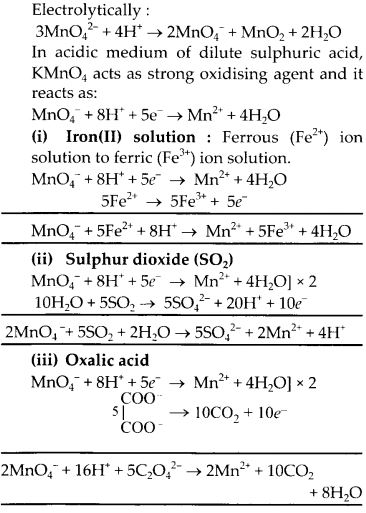Question 17.
For M2+/M and M3+/M2+ systems the P values for some metals are as follows

Use this data to comment upon

1. the stability of Fe3+ in acid solution as compared to that of Cr3+ or Mn3+ and
2. the ease with which iron can be oxidised as compared to a similar process for either chromium or manganese metal.

Solution:

1. From the E° values for M3+/M2+ for Cr, Mn and Fe, it is very clear that Fe3+ is more stable than Mn3+ in an acidic medium, whereas less stable than Cr3+ because of the higher reduction potential in comparison to Cr3+/Cr2+ and lower reduction potential than Mn3+/Mn2+.
2. Reduction potential for Mn2+/Mn is most negative and therefore, it will be most easily oxidised and ease of getting oxidised will be Mn > Cr > Fe.

Question 18.
Predict which of the following will be coloured in aqueous solution? Ti3+, V3+, Cu+, Sc3+, Mn2+, Fe3+ and CO32+. Give reasons for each.

Solution:
The configuration of the given metal ions can be given as

Question 19.
Compare the stability of +2 oxidation state for the elements of the first transition series.
Solution:
The common oxidation state of 3d series elements is + 2 which arises due to participation of only 4s electrons. The tendency to show highest oxidation state increases from Sc to Mn, then decreases due to pairing of electrons in 3d subshell. Thus in the series Sc(II) does not exist, Ti(II) is less stable than Ti(IV). At the other end of the series, oxidation state of Zn is +2 only.

Question 20.
Compare the chemistry of actinoids with that of the lanthanoids with special reference to
(i) electronic configuration
(ii) atomic and ionic sizes
(iii) oxidation state and
(iv) chemical reactivity.
Solution:
(i)Electronicconfiguration : Lanthanoids have general electronic configuration of [Xe] 4f1-14 5d0-1 6s2 and actinoids have general electronic configuration of [Rn]5f1-14 6d0-1 7s2. Thus, lanthanoids belong to 4 f-series whereas actinoids belong to 5 f-series.

(ii) Atomic and ionic sizes : The atomic size of lanthanoids decreases from lanthanum to lutetium. Though the decrease is not regular, in case of atomic radii, the decrease in the ionic size (M3+) is regular. Decrease in size between two successive elements is higher in actinoids due to poor screening by 5f electrons.

(iii) Oxidation state : The most common oxidation state of lanthanoids is +3 while actinoids show more variable oxidation states than lanthanoids ranging from +3 to +7. The tendency of showing greater range of oxidation states can be attributed to the fact that the 5f 6d and 7s levels are of comparable energies and larger distance of 5fas compared to 4f from the nucleus.

(iv) Chemical reactivity : Actinoids are far more reactive than lanthanoids. They react with non-metals at moderate temperatures whereas lanthanoids react at high temperatures. Most actinoids are attacked by HCl but are slightly affected by HNO3 due to formation of a protective layer of oxide and alkalies give no reaction.

Lanthanoids liberate hydrogen from dilute acids and burn in halogens to form halides.

Question 21.
How would you account for the following?
(i) Of the d4 species, Cr2+ is strongly reducing while manganese(lll) is strongly oxidising.
(ii) Cobalt(ll) is stable in aqueous solution but in the presence of complexing reagents it is easily oxidised.
(iii) The d1 configuration is very unstable in ions.
Solution:
(i) Both Cr2+ and Mn3+ have d4 configuration, Cr2+ is reducing since its configuration is converted to d3 from d4. d3 has half filled t28, configuration with higher stability. Mn3+ is oxidising since in changing from d4 to d5 the configuration becomes half filled which has extra stability,

(ii) Co(II) gets oxidised to Co(III) in presence of complexing agent because Co(III) is more stable than Co(II). Most of the strong field ligands cause pairing of electrons forming diamagnetic octahedral complexes which are very stable due to very large crystal field stabilization energy.

(iii) d1 configuration is very unstable in ions because after losing one more electron it will become more stable due to vacant d-orbital. All elements with d1 configuration are either reduced or undergo disproportionation, e.g.,

Question 22.
What is meant by ‘disproportionation’? Give two examples of disproportionation reaction in aqueous solution.
Solution:
Disproportionation reaction involves the oxidation and reduction of the same substance. The two examples of disproportionation reaction are

1. 2Cu+ → Cu2+ + Cu
2. 3MnO42- + 4H+ → 2MnO4 + MnO2 + 2H2O

Question 23.
Which metal in the first series of transition metals exhibits +1 oxidation state most frequently and why?
Solution:
Copper exhibits +1 oxidation state in the first series of transition metals because when one electron is lost, the configuration becomes stable due to fully filled d10 configuration.

Question 24.
Calculate the number of unpaired electrons in the following gaseous ions : Mn3+, Cr3+, V3+ and Ti3+. Which ono of these is the most stable in aqueous solution ?

Solution: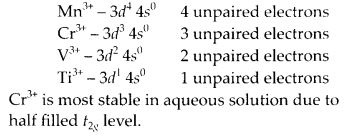Question 25.
Give examples and suggest reasons for the following features of the transition metal chemistry :
(i) The lowest, oxide of transition metal is basic, the highest is amphoteric/acidic.
(ii) A transition metal exhibits highest oxidation state in oxides and fluorides.
(iii) The highest oxidation state is exhibited in oxoanions of a metal.
Solution:
(i) Lowest oxidation compounds of transition metals are basic due to their ability to get oxidised to higher oxidation states. Whereas the higher oxidation state of metal and compounds gets reduced to lower ones and hence acts as acidic in nature.
e.g. MnO is basic whereas Mn2O7 is acidic.

(ii) Due to high electronegativities of oxygen and fluorine, the oxides and fluorides of transition metals exhibit highest oxidation state.
e.g. OSF6,V2O5

(iii) In oxoanions of metals, the metals from bonds with oxygen and hence are present in their highest oxidation states. For example : Cr forms CrO42- and Cr2O72-, both contain chromium in +6 oxidation state.

Permanganate ion, MnO4 contains Mn in its highest oxidation state of +7.

Question 26.
Indicate the steps in the preparation of

1. K2Cr2O7 from chromite ore
2. KMnO4 from pyrolusite ore.

Solution: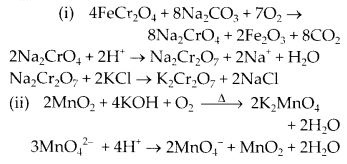Question 27.
What are alloys? Name an important alloy which contains some of the lanthanoid metals. Mention its uses.
Solution:
An allov is a blend of metals prepared by mixing the components. Alloys are homogeneous solid solutions in which the atoms of one metal are distributed randomly among the atoms of other. Misch metal is an alloy which contains some of the lanthanoid metals. It contains 95% lanthanoid metals, 5% iron and traces of S, C, Ca and Al. Mischmetall is used in Mg-based alloy to produce bullets, shell and lighter flint.

Question 28.
What are inner transition elements? Decide which of the following atomic numbers are the atomic numbers oftheinnertransition element: 29, 59, 74, 95,102,104.
Solution:
Lanthanoids and actinoids are called inner transition elements because inner f-orbitals are progressively filled and the last electron goes to anti penultimate f orbital.

Elements with atomic number 59, 95, 102 are inner transition metals because they belong to lanthanoids and actinoids.Question 29.
The chemistry of the actinoid elements is not so smooth as that of the lanthanoids. Justify this statement by giving some examples from the oxidation state of these elements.
Solution:
The actinoids are radioactive elements and the earlier members have relatively long half-lives, the latter ones have half-life values ranging from a day to 3 minutes for lawrencium (Z = 103). The latter members could be prepared only in nanogram quantities. These facts render their study more difficult.

There is a greater range of oxidation states, which is attributed to the fact that the 5f, 6d and 7s levels are of comparable energies. The actinoids show in general +3 oxidation state. The elements, in the first half of the series frequently exhibit higher oxidation states. For example, the maximum oxidation state increases from +4 in Th to +5, +6 and +7 respectively in Pa, U and Np but decreases in succeeding elements, The actinoids resemble the lanthanoids in having more compounds in +3 state than in the +4 state. However, +3 and +4 ions tend to hydrolyse. Because the distribution of oxidation states among the actinoids is so uneven and so different for the earlier and latter elements. It is unsatisfactory to review their chemistry in terms of oxidation states.

Question 30.
Which is the last element in the series of the actinoids? Write the electronic configuration of this element. Comment on the possible oxidation state of this element.
Solution:
Lawrencium (Lr) is the last element of actinoids. Its outer electronic configuration is 5f14 6d1 7s2 and its possible oxidation state is +3.

Question 31.
Use Hund’s rule to derive the electronic configuration of Ce3+ ion, and calculate its magnetic moment on the basis of ‘spin-only’ formula.

Solution:
The electronic configuration of Ce3+ is 4f1Question 32.
Name the members of the lanthanoid series which exhibit +4 oxidation state and those which exhibit +2 oxidation state. Try to correlate this type of behaviour with the electronic configurations of these elements.
Solution:
All lanthanoids show Ln3+ oxidation state. But some of them show +2 and +4 states also in solution and solid form like Ce4+, Eu2+, Yb2+, Tb4+, etc. The variable oxidation state is related to electronic configuration due to extra stability of half filled, fully filled or empty orbitals, e.g., Ce4+ has 4f0, Eu2+ has 4f7 Tb4+ has 4f7 and Yb2+ has 4f14 configuration.

Question 33.
Compare the chemistry of the actinoids with that of lanthanoids with reference to (i) electronic configuration (ii) oxidation states and (iii) chemical reactivity.
Solution:

Question 34.
Write the electronic configurations of the elements with the atomic numbers 61,91,101, and 109.

Solution:Question 35.
Compare the general characteristics of the first series of transition metals with those of the second and third series metals in the respective vertical columns. Give special emphasis on the following points
(i) electronic configurations
(ii) oxidation states
(iii) ionisation enthalpies and
(iv) atomic sizes.
Solution:
(i) Electronic configurations : In 1st transition series, 3d-orbitals are progressively filled whereas in 2nd transition series, 4d-orbitals are progressively filled and in 3rd transition series, 5d-orbitals are progressively filled.

(ii) Oxidations states : Elements show variable oxidation states in both the series. The highest oxidation state is equal to total number of electrons in ‘s’ as well as ‘d’ orbitals. The number of oxidation states shown are less in 5d transition series than 4d series. In 3d series +2 and +3 oxidation states are common and they form stable complexes in these oxidation states. In other series OsO4 and PtF6 are formed which are quite stable in higher oxidation state.

(iii) Ionisation enthalpies : The ionisation enthalpies in each series generally increases gradually from left to right. The ionisation enthalpy of 5d series do not differ appreciably due to lanthanoid contraction.

(iv) Atomic sizes : The atomic sizes of 4d and 5d-series do not differ appreciably due to lanthanoid contraction. The atomic radii of second and third series are larger than 3d series.

Question 36.
Write down the number of 3d electrons in each of the following ions: Ti2+, V2+, Cr3+, Mn2+, Fe2+, Fe3+, CO2+, Ni2+ and Cu2+. Indicate how would you expect the five 3d orbitals to be occupied for these hydrated ions (octahedral).

Solution: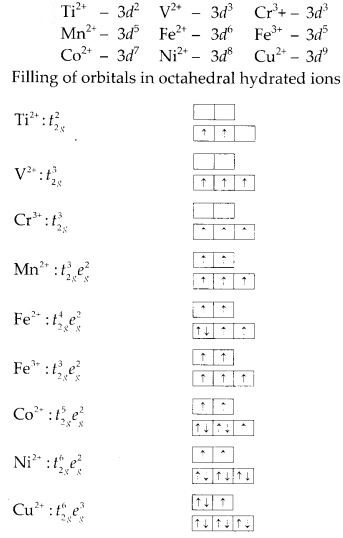Question 37.
Comment on the statement that elements of the first transition series possess many properties different from those of heavier transition elements.
Solution:

1. In first transition series lower oxidation state is more stable whereas in heavier transition elements higher oxidation states are more stable.
2. The ionisation enthalpy of 5d transition series is higher than 3d and 4d transition series.
3. M-M bonding is most common in heavier transition metals but less in first series.
4. The elements of first transition series do not form complexes with higher coordination number of 7 and 8.
5. The elements of first series can form high spin or low spin complexes depending upon strength of ligands but elements of other series form low spin complexes irrespective of strength of ligands.

Question 38.
What can be inferred from the magnetic moment values of the following complex species?

Question 38.
What can be inferred from the magnetic moment values of the following complex species?

Solution: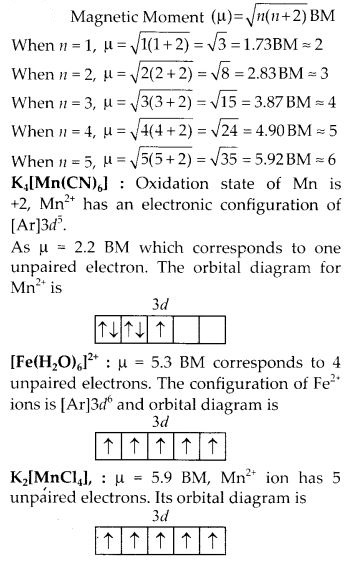All Chapter NCERT Solutions For Class12 Chemistry

—————————————————————————–

All Subject NCERT Solutions For Class12

*************************************************

Remark:

I think you got complete solutions for this chapter. If You have any queries regarding this chapter, please comment on the below section our subject teacher will answer you. We tried our best to give complete solutions so you got good marks in your exam.

If these solutions have helped you, you can also share Careerkundali.in to your friends.

Best of Luck!!